Courses

Test: DFT Properties

10 Questions MCQ Test Digital Signal Processing | Test: DFT Properties

Description
Attempt Test: DFT Properties | 10 questions in 10 minutes | Mock test for Electrical Engineering (EE) preparation | Free important questions MCQ to study Digital Signal Processing for Electrical Engineering (EE) Exam | Download free PDF with solutions
QUESTION: 1

If X(k) is the N-point DFT of a sequence x(n), then what is the DFT of x*(n)?

Solution:

According to the complex conjugate property of DFT, we have if X(k) is the N-point DFT of a sequence x(n), then what is the DFT of x*(n) is X*(N-k).

QUESTION: 2

If X(k) is the N-point DFT of a sequence x(n), then circular time shift property is that N-point DFT of x((n-l))N is X(k)e-j2πkl/N.

Solution:

Explanation: According to the circular time shift property of a sequence, If X(k) is the N-point DFT of a sequence x(n), then the N-pint DFT of x((n-l))N is X(k)e-j2πkl/N.

QUESTION: 3

What is the circular convolution of the sequences x1(n)={2,1,2,1} and x2(n)={1,2,3,4}, find using the DFT and IDFT concepts?

Solution:

Explanation: Given x1(n)={2,1,2,1}=>X1(k)=[6,0,2,0] Given x2(n)={1,2,3,4}=>X2(k)=[10,-2+j2,-2,-2-j2] when we multiply both DFTs we obtain the product
X(k)=X1(k).X2(k)=[60,0,-4,0] By applying the IDFT to the above sequence, we get
x(n)={14,16,14,16}.

QUESTION: 4

What is the circular convolution of the sequences x1(n)={2,1,2,1} and x2(n)={1,2,3,4}?

Solution:

Explanation: We know that the circular convolution of two sequences is given by the expression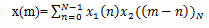For m=0,x2((-n))4={1,4,3,2}
For m=1,x2((1-n))4={2,1,4,3}
For m=2,x2((2-n))4={3,2,1,4}
For m=3,x2((3-n))4={4,3,2,1}
Now we get x(m)={14,16,14,16}.

QUESTION: 5

If x1(n),x2(n) and x3(m) are three sequences each of length N whose DFTs are given as X1(k),X2(k) and X3(k) respectively and X3(k)=X1(k).X2(k), then what is the expression for x3(m)?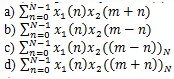Solution:

Explanation: If x1(n),x2(n) and x3(m) are three sequences each of length N whose DFTs are given as X1(k),X2(k) and X3(k) respectively and X3(k)=X1(k).X2(k), then according to the multiplication property of DFT we have x3(m) is the circular convolution of x1(n) and x2(n).

QUESTION: 6

. If x(n) is real and odd, then what is the IDFT of the given sequence?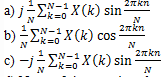d) None of the mentioned

Solution:

Explanation: If x(n) is real and odd, that is x(n)=-x(N-n), then XR(k)=0. Hence X(k) is purely imaginary and odd. Since XR(k) reduces to zero, the IDFT reduces to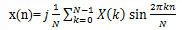QUESTION: 7

If x(n) is real and even, then what is the DFT of x(n)?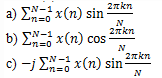d) None of the mentioned

Solution:

Explanation: Given x(n) is real and even, that is x(n)=x(N-n)
We know that XI(k)=0. Hence the DFT reduces to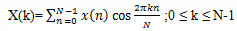QUESTION: 8

If x(n) is a real sequence and X(k) is its N-point DFT, then which of the following is true?

Solution:

Explanation: We know that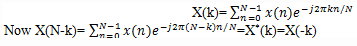Therefore,
X(N-k)=X*(k)=X(-k)

QUESTION: 9

If x(n) is a complex valued sequence given by x(n)=xR(n)+jxI(n), then what is the DFT of xR(n)?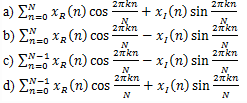Solution:

Explanation: Given x(n)=xR(n)+jxI(n)=> xR(n)=1/2(x(n)+x*(n))
Substitute the above equation in the DFT expression
Thus we get,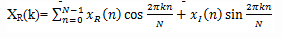QUESTION: 10

If X1(k) and X2(k) are the N-point DFTs of x1(n) and x2(n) respectively, then what is the N-point DFT of x(n)=ax1(n)+bx2(n)?

Solution:

Explanation: We know that, the DFT of a signal x(n) is given by the expression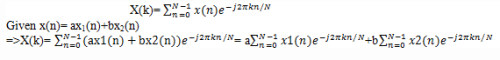=>X(k)= aX1(k)+bX2(k).Use Code STAYHOME200 and get INR 200 additional OFF Use Coupon Code

Track your progress, build streaks, highlight & save important lessons and more!

Similar ContentRelated tests